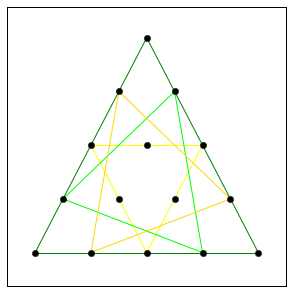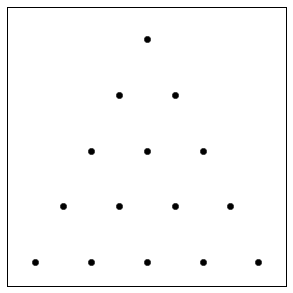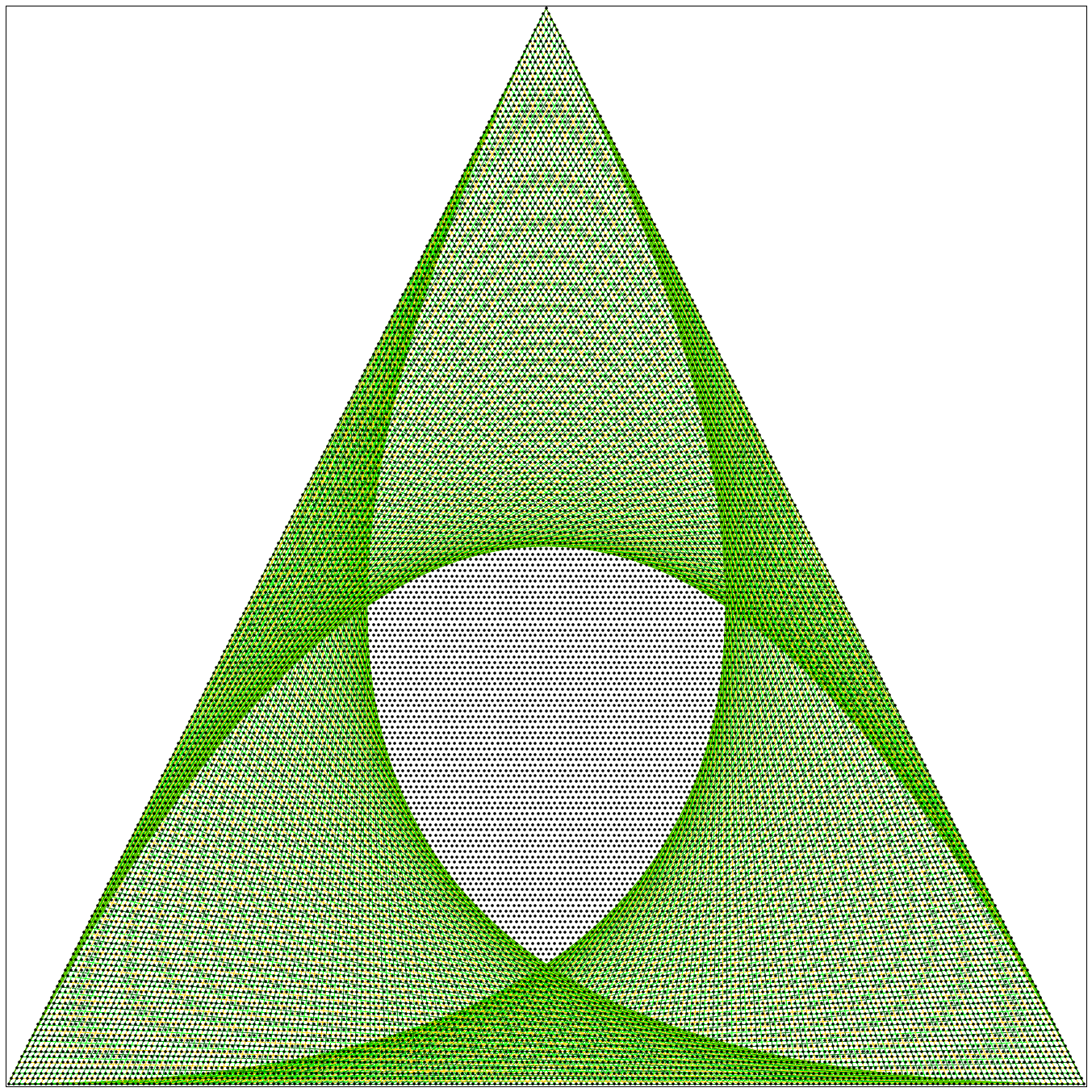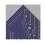# "Too Many Triangles?!":General Solution and ThoughtsThis note is for the question Too may Triangles?!

Consider an equilateral triangular lattice like the one below :We'll say that this specific lattice is of size 5 since it has 5 dots on its outer perimeter.

Here we'll think about the question :

On an equilateral triangular lattice of size $n$, by joining dots with straight lines, how many different equilateral triangles can we make?

Part 1 :The Answer and a Solution

Here we'll show that the answer is ${n+2 \choose 4} = \frac{n(n+2)(n^2-1)}{24}.$

We'll define a new $i$ equilateral triangle to be an equilateral triangle that can be made on a equilateral triangular lattice of size $i$ but not in any smaller equilateral triangular lattice. For example, here are all the new $5$ equilateral triangles we can make :Clearly there exist $i-1$ amount of new $i$ equilateral triangle in an equilateral triangular lattice of size $i$ for all natural numbers $i$.

So how many unique equilateral triangular lattice of size $i$ can we find in an equilateral triangular lattice of size $n$ where $n \ge i$?

Well we can find $n+1 -i$ in the $1^{st}$ row (the bottom row) and $n+1 -i -1$ in the $2^{nd}$ row and in general we have $n+1 -i - k$ in the $k^{th}$ row for $k \le n-i$, so the total is given by $\sum_{k=1}^{k=n-i+1} k = \frac{(n-i+1)(n+2-i)}{2}$ by the sum of consecutive integers formula.

So the total amount of equilateral triangles is given by

the number of new $i$ equilateral triangle $\times$ the number of unique equilateral triangular lattice of size $i$ can we find in an equilateral triangular lattice of size $n$ which is the same as

\begin{aligned} &\sum_{i=1}^{i = n} (i-1)\times \frac{(n-i+1)(n+2-i)}{2} \\ & \text{and using the substitution \j = i-1\, we have } \\=& \sum_{j=0}^{j = n-1} j\times \frac{(n-j)(n-j+1)}{2} = \sum_{j=1}^{j = n} j\times \frac{(n-j)(n-j+1)}{2} \\ =&\frac{1}{2} \sum_{j=1}^{j = n}j^3 -j^2(2n+1) + j(n(n+1)) \\ =& \frac{1}{2}[\frac{n^2(n+1)^2}{4} + \frac{n(n+1)(2n+1)^2}{6} + \frac{n^2(n+1)^2}{2}] \\=&\frac{n(n+1)}{24}[3n(n+1) + 2(2n+1)^2 - 6n(n+1)] \\=&\frac{n(n+1)}{24}[n^2+n-2] \\=&\frac{(n+1)(n)(n-1)(n+2)}{24} = {n+2 \choose 4}. \end{aligned}

Part 2 : Observations and Questions

Let

• $T_n =$ The total number of Equilateral Triangles we can form on an equilateral triangular lattice of size $n$ = ${n+2 \choose 4}.$

• $D_n =$ The total number of Equilateral Triangles we can form on an equilateral triangular lattice of size $n$ only using lines that are not parallel to the sides of the largest equilateral triangle on the perimeter of the lattice = ${n+1 \choose 3}$ .

• $S_n =$ The total number of Equilateral Triangles we can form on an equilateral triangular lattice of size $n$ only using lines that are parallel to the sides of the largest equilateral triangle on the perimeter of the lattice $= {n+1 \choose 4}$ .

Note that $D_n = {n+1 \choose 3}$ correspond to the fourth diagonal line of Pascal's Triangle containing the entries of $1,4,10,20,35,56...$. We can interpret these as the number of points needed to describe a tetrahedron of "size n+1".

Similarly, $T_n = {n+2 \choose 4}$ and $S_n = {n+1 \choose 4}$ corresponds to the fifth diagonal line of Pascal's Triangle containing the entries $1,5,15,35,70,126,210...$. We can interpret this row as "The number of points we need to describe a fourth dimensional triangle (aka a pentatope) of "size" n+2 and n+1 respectively" .

It is true that $T_n = {n+2 \choose 4} = {(n+1) + 1 \choose 4} = D_{n+1}$ which means that the total number of "non parallel equilateral triangles" in an $n$ sized lattice is the same as the total number of equilateral triangles we can make in an $n-1$ sized lattice.

Is there a more intuitive reason why? This also true for the analogue square question.

Note that as $n \rightarrow \infty$ the area enclosed by the new n equilateral triangles seems to tend to a Releaux Triangle. In this case $n = 200.$and seems to have similar results for square analogue. $\square$Note by Roberto Nicolaides
6 years, 4 months ago

This discussion board is a place to discuss our Daily Challenges and the math and science related to those challenges. Explanations are more than just a solution — they should explain the steps and thinking strategies that you used to obtain the solution. Comments should further the discussion of math and science.

When posting on Brilliant:

• Use the emojis to react to an explanation, whether you're congratulating a job well done , or just really confused .
• Ask specific questions about the challenge or the steps in somebody's explanation. Well-posed questions can add a lot to the discussion, but posting "I don't understand!" doesn't help anyone.
• Try to contribute something new to the discussion, whether it is an extension, generalization or other idea related to the challenge.

MarkdownAppears as
*italics* or _italics_ italics
**bold** or __bold__ bold
- bulleted- list
• bulleted
• list
1. numbered2. list
1. numbered
2. list
Note: you must add a full line of space before and after lists for them to show up correctly
paragraph 1paragraph 2

paragraph 1

paragraph 2

[example link](https://brilliant.org)example link
> This is a quote
This is a quote
    # I indented these lines
# 4 spaces, and now they show
# up as a code block.

print "hello world"
# I indented these lines
# 4 spaces, and now they show
# up as a code block.

print "hello world"
MathAppears as
Remember to wrap math in $$ ... $$ or $ ... $ to ensure proper formatting.
2 \times 3 $2 \times 3$
2^{34} $2^{34}$
a_{i-1} $a_{i-1}$
\frac{2}{3} $\frac{2}{3}$
\sqrt{2} $\sqrt{2}$
\sum_{i=1}^3 $\sum_{i=1}^3$
\sin \theta $\sin \theta$
\boxed{123} $\boxed{123}$

Sort by:

Nicely explained Roberto :)

- 6 years, 4 months ago

Awesome! Hats off!!

- 6 years, 4 months ago

Thank you for the kind words :)

- 6 years, 4 months ago